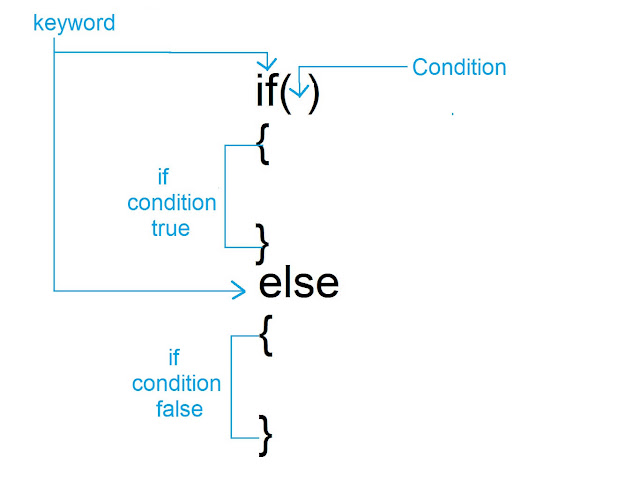# If else statement in C Language

Hello friends how are you, today in this blog i will teach you what is if else statement, syntax of if else, how if else statement works and many programs using if else statement in a very simple way.

Let's start

👉Syntax1.If the condition is true if part executes and if the condition is false else part executes.
2.In the case of if in the place of condition always zero and non-zero value is checked in which zero means condition false and non-zero means condition true

👉Example 1

```#include<stdio.h>
int main()
{
//Assigning value to the variable
int x=50,y=80;
//checking the condition
if(x>y)
{
printf("x is greater than y");
}
else
printf("y is greater than x");
}
/*
### Output ###
y is greater than x
*/
```

👉Example 2

```#include<stdio.h>
int main()
{
//Assigning value to the variable
int x=50,y=80;
//checking the condition
if(x<y)//condition is true here
{
printf("x is greater than y");
}
else
printf("y is greater than x");
}
/*
### Output ###
x is greater than y
*/
```

👉Example 3

```#include<stdio.h>
int main()
{
if(9)
{
printf("Hello");
}
else
printf("hi");
}
/*
### Output ###
Hello
*/
```
In case of if at the place of condition always zero and non-zero value is checked in which zero means condition false otherwise true. Here in above example 9 is a non-zero value so condition will be true and output will be Hello

👉Example 4

```#include<stdio.h>
int main()
{
if(0)
{
printf("Hello");
}
else
printf("hi");
}
/*
### Output ###
Hi
*/
```
In case of if at the place of condition always zero and non-zero value is checked in which zero means condition false otherwise true. Here in above example there is 0 in place of condition so condition will be false and output will be Hi

👉Example 5

```#include<stdio.h>
int main()
{
if(8,9,6,0,0,7,8)
{
printf("Hello");
}
else
{
printf("Hi");
}
}
/*
### Output ###
Hello
*/
```

👉Program: Check given number is even or odd

```#include<stdio.h>
int main()
{
int no;
printf("Enter any number:");
scanf("%d",&no);
if(no%2==0)
printf("even");
else
printf("odd");
}
/*
### Output ###
Enter any number:54
even
*/
```

Request:-If you found this post helpful then let me know by your comment and share it with your friend.

If you want to ask a question or want to suggest then type your question or suggestion in comment box so that we could do something new for you all.

If you have not subscribed my website then please subscribe my website.

Try to learn something new and teach  something new to other.
Thanks.